# Civil Engineering - Strength of Materials

### Exercise :: Strength of Materials - Section 3

41.

A joint of a frame is subjected to three tensile force P, Q and R equally inclined to each other. If P is 10 tonnes, the other forces will be

 A. Q = 10 tonnes and R = zero B. R + 10 tonnes and Q = zero C. Q + R = 10 tonnes D. Q - R = zero E. Q and R each is equal to 10 tonnes.

Explanation:

No answer description available for this question. Let us discuss.

42.

If a member is subjected to a tensile force P, having its normal cross-section A, the resulting shear stress in an oblique plane inclined at an angle θ to its transverse plane, is

 A.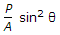B.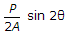C.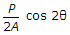D.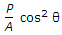E.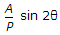Explanation:

No answer description available for this question. Let us discuss.

43.

A simply supported beam carries two equal concentrated loads W at distances L/3 from either support. The maximum bending moment

 A.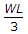B.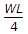C.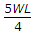D.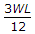E.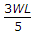Explanation:

No answer description available for this question. Let us discuss.

44.

If a solid shaft is subjected to a torque T at its end such that maximum shear stress does not exceed fz the diameter of the shaft will be

 A.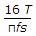B.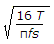C.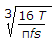D. none of these.

Explanation:

No answer description available for this question. Let us discuss.

45.

Pick up the correct statement from the following :

 A. The distance of the eccentric axial load from the C.G. beyond which tension develops, is known as kern distance B. In visco-elastic material, stress-strain relation is dependent on time C. An instropic material has different properties is different directions D. An orthotropic material has different properties in three mutually perpendicular directions E. All the above.